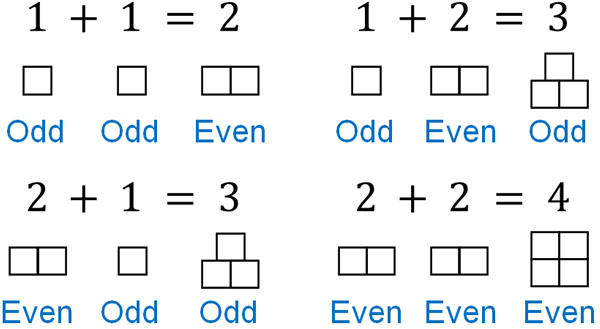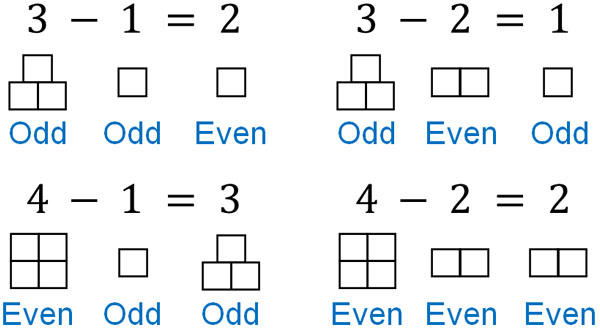In elementary school math, we study even numbers and odd numbers. So what are even numbers and odd numbers? And how can we distinguish between even and odd numbers?

The concepts of even and odd numbers are often used in our daily lives. For example, in programming, we use even and odd numbers. Also, we frequently use even and odd numbers when shopping.

Even numbers and odd numbers have properties. So, not only should we be able to distinguish between even and odd numbers, but we should also be able to figure out what the answer will be after addition, subtraction, and multiplication. We will explain how to distinguish between even and odd numbers and how to calculate them.

## What Are Even and Odd Numbers: Definition and Meaning

First of all, what is even and odd numbers, which are studied in elementary school math? By understanding the definitions of the words, you will be able to understand what kind of numbers they are.

Even and odd numbers are defined as follows.

• Even: a number that is divisible by two.
• Odd: a number that is not divisible by two.

If a number is divisible by 2, it is an even number. If a number is not divisible by 2, it is an odd number. For example, is 32 an even number or an odd number? As shown below, 32 can be divided by 2.

• $32÷2=16$

Therefore, 32 is an even number.

Also, since the number divisible by 2 is an even number, if the number increases by 2 for 2, it will always be an even number. In other words, multiples of 2 are even numbers.

• 2, 4, 6, 8, 10, 12, 14…

All of these numbers are even.

On the other hand, is 17 even or odd? 17 is not divisible by 2. The answer will have a remainder.

• $17÷2=8$ R $1$

Therefore, 17 is an odd number. You can distinguish if a number is even or odd by whether it is divisible by two or not. In other words, any number that increases by 2 for 1 is an odd number.

• 1, 3, 5, 7, 9, 11, 13…

All of these numbers are odd numbers. Both even and odd numbers increase by two.

### How to Distinguish and Remember Even and Odd Numbers

How can we effectively distinguish between even and odd numbers? As mentioned above, a number is even if it is divisible by two. Any other number is an odd number. In other words, multiples of two are even numbers. On the other hand, if it is not a multiple of two, it is an odd number.

So, let’s focus on the ones place. We can distinguish between even numbers and odd numbers as follows.

• Even numbers: 0, 2, 4, 6, and 8 in the ones place
• Odd numbers: 1, 3, 5, 7, and 9 in the ones place

No matter how big the number is, if the ones place is 0, 2, 4, 6, or 8, it is an even number. Also, no matter how big the number is, if the ones place is 1, 3, 5, 7, or 9, it is an odd number. You can determine whether a number is even or odd by checking the ones place. It doesn’t matter if the number in the tenth or hundredth place is even or odd.

For example, is 395374 an even number or an odd number? If you check 395374, you will see that the ones place is 4. 4 is an even number, so 395374 is an even number. In fact, 395374 can be divided by 2.

• $395374÷2=197687$

To see if the number is even or odd, check the ones place.

## Is the Answer to Addition or Subtraction an Even or Odd Number?

Next, let’s consider whether adding or subtracting will result in an answer that is even or odd. When we do addition, the following properties exist.

• Odd + Odd = Even number
• Odd + Even = Odd number
• Even + Odd = Odd number
• Even + Even = Even number

When explaining the reason, we have to prove it using junior high school mathematics. Therefore, we will omit the reason, but it will always look like the above. So, let’s consider simple integers as follows.On the other hand, what happens in subtraction? As with addition, subtraction yields the same result, as follows.

• Odd – Odd = Even number
• Odd – Even = Odd number
• Even – Odd = Odd number
• Even – Even = Even numberWhen considering even and odd numbers, if numbers with the same properties (odd and odd, or even and even) are added or subtracted, the answer will be even. On the other hand, when numbers with different properties (even and odd) are added or subtracted, the answer will be odd. This property is also true for large numbers.

### In Multiplication, Is the Answer an Even or Odd Number?

What happens in multiplication? In multiplication, if you multiply by an even number, the answer will always be an even number. If you learned about multiples in elementary school math, you would understand why this happens.

As mentioned above, a number that is divisible by two is an even number. An even number is also a multiple of 2. When multiplying by 2, of course, the answer will always be a multiple of 2.

• $1×\textcolor{red}{2}=2$: even
• $2×\textcolor{red}{2}=4$: even
• $3×\textcolor{red}{2}=6$: even
• $4×\textcolor{red}{2}=8$: even

Also, if you multiply not only by 2 but also by an even number, the answer will always be an even number. This is because an even number is a multiple of 2.

• $3×\textcolor{red}{4}=12$: even
• $3×\textcolor{red}{6}=24$: even
• $5×\textcolor{red}{8}=40$: even
• $6×\textcolor{red}{10}=60$: even

The only case where the answer is odd in multiplication is when odd numbers are multiplied together. As shown below, when multiplying an odd number by an odd number, the answer is an odd number.

• $1×3=3$: odd
• $5×7=35$: odd
• $3×9=27$: odd
• $7×11=77$: odd

So, in multiplication, we get the following.

• Odd × Odd = Odd number
• Odd × Even = Even number
• Even × Odd = Even number
• Even × Even = Even number

Unlike addition and subtraction, multiplication has a high probability of the answer being an even number. In order for the answer to be odd, the odd numbers must be multiplied together.

## Understanding the Properties of Even and Odd Numbers

One of the most frequently used concepts is even and odd numbers. Since there are many situations in which we use even and odd numbers in our daily lives, it is important to understand how to distinguish between even and odd numbers.

If a number is divisible by 2, it is an even number. Also, if you divide by 2 and get the remainder, the number is odd. One way to determine whether a number is even or odd is to look at the ones place. If you check the ones place, you will be able to distinguish whether it is an even number or an odd number.

In addition, understand what the answer will be when you do addition or subtraction. When you add or subtract two even numbers or two odd numbers, the answer will be an even number. On the other hand, when an even number and an odd number are used for addition or subtraction, the answer will be an odd number.

Understand these properties. If you do not understand even and odd numbers, you will have trouble in your daily life. As adults, we use even and odd numbers in many situations, so learn these definitions and concepts.